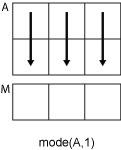# mode

## 语法

``M = mode(A)``
``M = mode(A,'all')``
``M = mode(A,dim)``
``M = mode(A,vecdim)``
``[M,F] = mode(___)``
``[M,F,C] = mode(___)``

## 说明

````M = mode(A)` 返回 `A` 的样本众数，即 `A` 中出现次数最多的值。如果有多个值以相同的次数出现，`mode` 将返回其中最小的值。对复杂的输入，最小值是排序列表的第一个值。 如果 `A` 为向量，则 `mode(A)` 返回 `A` 中出现次数最多的值。如果 `A` 为非空矩阵，那么 `mode(A)` 将返回包含 `A` 每列众数的行向量。如果 `A` 为 0×0 空矩阵，`mode(A)` 返回 `NaN`。如果 `A` 为多维数组，则 `mode(A)` 将沿大小不等于 `1` 的第一个数组维度的值视为向量，并返回一个由出现次数最多的值组成的数组。此维度的大小将变为 `1`，而所有其他维度的大小保持不变。 ```

``M = mode(A,'all')` 计算 `A` 的所有元素的众数。此语法适用于 MATLAB® R2018b 及更高版本。`

``M = mode(A,dim)` 返回维度 `dim` 上的元素的模式。例如，如果 `A` 为矩阵，则 `mode(A,2)` 是包含每一行的出现次数最多值的列向量。`

``M = mode(A,vecdim)` 计算向量 `vecdim` 所指定的维度上的众数。例如，如果 `A` 是矩阵，则 `mode(A,[1 2])` 是 `A` 中所有元素的众数，因为矩阵的每个元素都包含在由维度 1 和 2 定义的数组切片中。`

``[M,F] = mode(___)` 还会结合前面语法中的任意输入参数，返回一个频率数组 `F`。`F` 与 `M` 大小相同，而且 `F` 的每个元素代表 `M` 中对应元素的出现次数。`

``[M,F,C] = mode(___)` 返回与 `M` 和 `F` 同样大小的元胞数组 `C`。`C` 中的每个元素都是与 `M` 中对应元素出现频率相同的所有值的排序向量。`

## 示例

`A = [3 3 1 4; 0 0 1 1; 0 1 2 4]`
```A = 3×4 3 3 1 4 0 0 1 1 0 1 2 4 ```

`M = mode(A)`
```M = 1×4 0 0 1 4 ```

`A = [3 3 1 4; 0 0 1 1; 0 1 2 4]`
```A = 3×4 3 3 1 4 0 0 1 1 0 1 2 4 ```

`M = mode(A,2)`
```M = 3×1 3 0 0 ```

```rng('default') A = randi(10,[1,3,4])```
```A = A(:,:,1) = 9 10 2 A(:,:,2) = 10 7 1 A(:,:,3) = 3 6 10 A(:,:,4) = 10 2 10 ```

`M = mode(A)`
```M = M(:,:,1) = 2 M(:,:,2) = 1 M(:,:,3) = 3 M(:,:,4) = 10 ```

```M = mode(A,1); isequal(A,M)```
```ans = logical 1 ```

```A(:,:,1) = [2 4; 2 1]; A(:,:,2) = [6 2; 3 3]; A(:,:,3) = [4 4; 7 4]; M1 = mode(A,[1 2])```
```M1 = M1(:,:,1) = 2 M1(:,:,2) = 3 M1(:,:,3) = 4 ```

`M2 = mode(A,[1 2 3])`
```M2 = 4 ```
`Mall = mode(A,'all')`
```Mall = 4 ```

`A = [3 3 1 4; 0 0 1 1; 0 1 2 4]`
```A = 3×4 3 3 1 4 0 0 1 1 0 1 2 4 ```

`[M,F] = mode(A)`
```M = 1×4 0 0 1 4 ```
```F = 1×4 2 1 2 2 ```

`F(1)``2`，因为 `M(1)` 在第一列中出现两次。

`A = [3 3 1 4; 0 0 1 1; 0 1 2 4]`
```A = 3×4 3 3 1 4 0 0 1 1 0 1 2 4 ```

`[M,F,C] = mode(A,2)`
```M = 3×1 3 0 0 ```
```F = 3×1 2 2 1 ```
```C=3×1 cell array {[ 3]} {2x1 double} {4x1 double} ```

`C{2}` 为 2×1 向量 `[0;1]`，因为第二行中值 `0``1` 出现次数为 `F(2)`

`C{3}` 为 4×1 向量 `[0;1;2;4]`，因为第三行中所有值出现次数为 `F(3)`

```rng('default') A = randi(10,[1,4],'uint16')```
```A = 1x4 uint16 row vector 9 10 2 10 ```

`[M,F] = mode(A)`
```M = uint16 10 ```
```F = 2 ```
`class(M)`
```ans = 'uint16' ```

`M` 与输入 `A` 的类相同。

## 输入参数

• 如果 `dim = 1`，则 `mode(A,1)` 返回包含每一列中出现次数最多元素的行向量。• 如果 `dim = 2`，则 `mode(A,2)` 返回包含每一列中出现次数最多元素的列向量。## 输出参数

`M` 的类与输入数组 `A` 的类相同。

`F` 的类总是 `double`

## 提示

• `mode` 函数对于离散数据或粗略舍入数据非常有用。一个连续概率分布的众数被定义为其密度函数的峰值。将 `mode` 函数应用到不太可能产生良好峰值估计值的分布样本；这样能更好地计算出直方图或密度估计值并计算估计值的峰值。此外，`mode` 函数也不适合查找具有多个众数的分布的峰值。